+

# Venn Diagrams

Author: Sophia Tutorial
##### Description:

Identify elements in a Venn diagram.

(more)

Sophia’s self-paced online courses are a great way to save time and money as you earn credits eligible for transfer to many different colleges and universities.*

No credit card required

37 Sophia partners guarantee credit transfer.

299 Institutions have accepted or given pre-approval for credit transfer.

* The American Council on Education's College Credit Recommendation Service (ACE Credit®) has evaluated and recommended college credit for 32 of Sophia’s online courses. Many different colleges and universities consider ACE CREDIT recommendations in determining the applicability to their course and degree programs.

Tutorial
what's covered
This tutorial will cover the topic of Venn diagrams. Our discussion breaks down as follows:

1. Venn Diagrams
2. Complements in a Venn Diagram
3. Venn Diagrams with no Overlap

## 1. Venn Diagrams

Venn diagrams are an important idea in probability theory. Venn diagrams were invented by the logician John Venn. They're useful for understanding the relationships between two different sets of objects or two different events in probability.

A typical Venn diagram with two intersecting sets has four parts to it:

• The part that is only in event A, but not in event B.
• The part that is only in event B, but not in event A.
• The part that is both events A and B, where the two ovals intersect.
• The part that is neither A nor B--the part outside the intersecting ovals.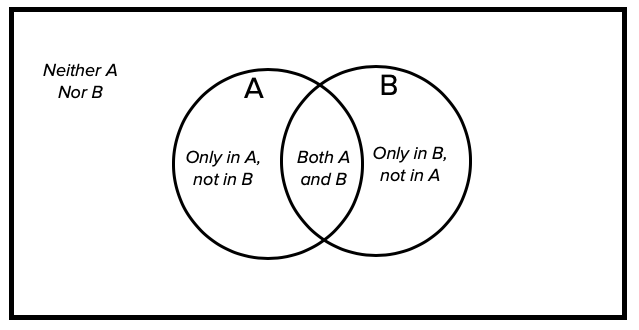EXAMPLE

Suppose that in a high school of 80 students, 18 of them take statistics, 15 take economics, and 8 students take both.

What would the Venn diagram look like in terms of the number of students that go in each of these four areas?

The first instinct of most people is to put 18 in the statistics bubble, 15 in the economics bubble, 8 in the intersection of both bubbles, and whatever is left of the 80 on the outside. That graph would look like this: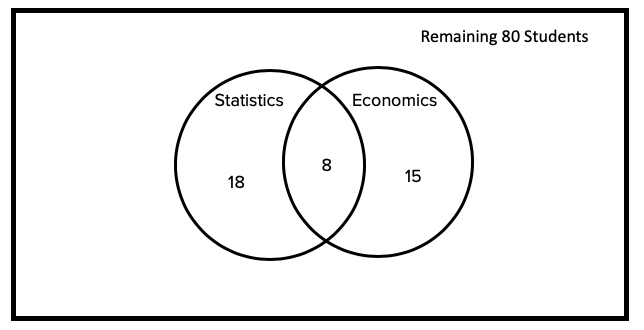That's not a terrible idea, but it has a few problems. Start with the innermost portion of the circle, the 8 students that take both statistics and economics. Recall that 15 students take economics. However, 8 of those students are also taking statistics, and they fall into the middle category. Therefore, of the 15 total students taking economics, 8 of them should be in the center category, and the remaining 7 should be in the "economics only" category.
Apply the same logic to the other side. Of the 18 students taking statistics, 8 are also taking economics, which means there are 10 students taking statistics only.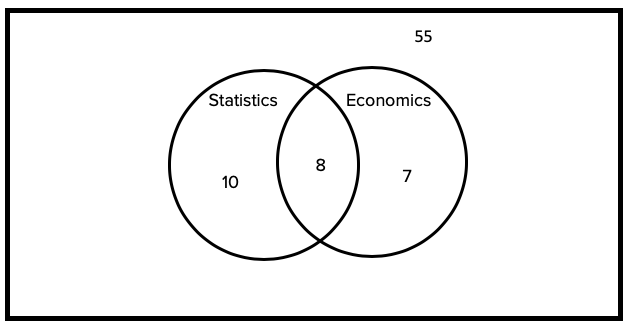If there are 10 students in just statistics, 7 students in just economics, and 8 in both, this is a total of 25 students. This tells us that, of the 80 students, 55 of them are taking neither statistics nor economics.
term to know

Venn Diagram
A way to compare and contrast events from a chance experiment. Two events may both occur, only one or the other may occur, or neither may occur.

## 2. Complements in a Venn Diagram

We can also show complements in a Venn diagram. Complements are everything that's not in a particular event.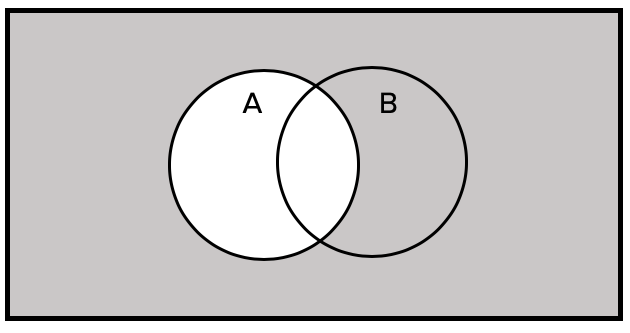The complement of A is everything outside of the A bubble, including the part of B that does not overlap with A.

The overlapping portion is in A, so it is therefore not part of the complement.

## 3. Venn Diagrams with no Overlap

Not all Venn diagrams need overlap like this. Sometimes, the two events, A and B, don't have any outcomes in common, which looks like this:

EXAMPLE

Suppose A is the event of rolling an even number and B is the event rolling a five. There's no overlap between the two ideas, so you can draw the bubbles as separate. In the "neither" category would be any rolls that are odd but not five, which leaves one and three.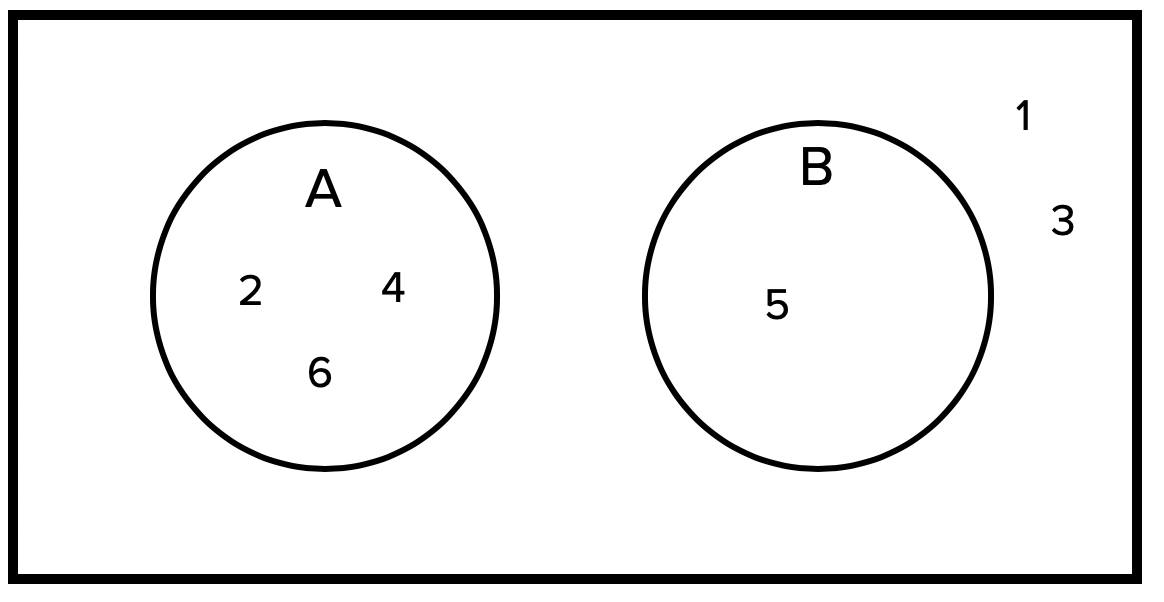summary
Many relationships between events can be represented using Venn diagrams: overlap, complements, and not overlapping. Venn diagrams are also useful for probability. Two-event Venn diagrams, such as the ones in this tutorial, consist of four areas: the A-only area, the B-only area, the both A and B area, and the neither A nor B area. The total number of items can be found by adding up all the subcategories within that event.

Good luck!

Source: Adapted from Sophia tutorial by Jonathan Osters.

Terms to Know
Venn Diagram

A way to compare and contrast events from a chance experiment. Two events may both occur, only one or the other may occur, or neither may occur.

Rating